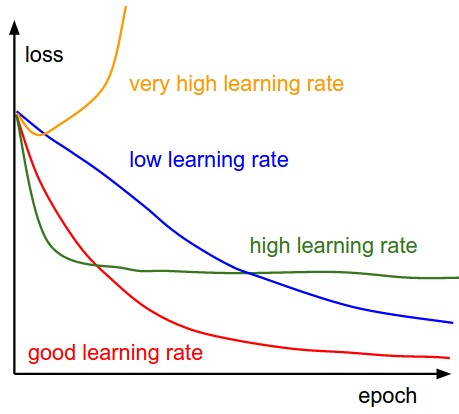# What is a model learning rate? Is a high learning rate always good?

The learning rate is a hyperparameter that plays a crucial role in the training of a neural network. It determines the step size at which the optimizer makes updates to the weights of the network during training. The learning rate is often represented as a scalar value, and it is typically chosen before training begins.

In general, a higher learning rate means that the optimizer will make larger updates to the weights, while a lower learning rate leads to smaller updates. The learning rate controls the speed at which the model learns, and it can have a significant impact on the performance of the model.

If the learning rate is set too high, the optimizer may overshoot the optimal weights and fail to converge. On the other hand, if the learning rate is set too low, the optimizer may get stuck in a local minimum or a plateau region and take a long time to reach the optimal weights.

In addition to affecting the convergence of the model, the learning rate can also impact the stability of the model. A high learning rate may cause the model to oscillate or diverge, while a low learning rate can lead to slow and stable learning.

To choose an appropriate learning rate, it is often necessary to experiment with different values and observe the results. There are also techniques such as learning rate schedules and adaptive learning rates that can help to automatically adjust the learning rate during training. It is important to carefully select the learning rate, as it can have a significant impact on the performance and convergence of the model.The learning rate determines the speed at which a model is able to reach its optimal accuracy, known as convergence to a local minimum. Setting the learning rate appropriately from the start can reduce the amount of time required for training the model.